# Cohesive Zone Modeling and Viscous RegularizationAugust 28, 2015 By: Michael Bak

Finite element analysis, like any numerical procedure, can exhibit convergence difficulties when trying to solve problems involving nonlinear effects. Often, the convergence issues in a structural analysis stem from a sudden change in stiffness, such as might occur when a contact element comes in or out of contact, or a constitutive law has a sudden change in the slope of the stress-strain curve. A particular example of a sudden change in stiffness that causes convergence difficulties is modeling delamination using the bilinear cohesive zone material law.

A cohesive zone material model predicts the behavior of an interface between layers, from elastic deformation, to softening and damage accumulation, ending in eventual delamination.  In finite elements, the interface is modeled with elements that are initially zero thickness but provide the connection between two layers.  A popular cohesive zone law, shown in Figure 1, is the bilinear traction-separation law. The initial slope represents the linear elastic material behavior of the interface. When the peak traction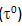is achieved at a separation of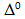, damage is initiated, and the traction-separation response is represented by the downward slope. This softening can eventually lead to the maximum separation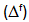,  at which point the interface is failed and can open freely.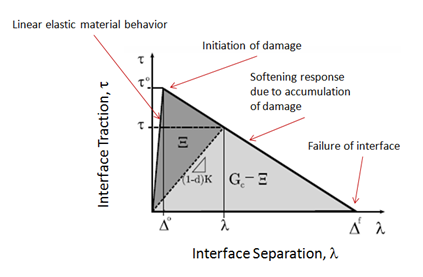The bilinear cohesive zone law uses a damage parameter (d) to define the current state of the interface.  At damage initiation, damage is zero, and as the separation increases, the damage will increase until it reaches a maximum value of one at failure.  At any point on the softening slope, the reduced stiffness of the damaged interface is equal to (1-d)*K, where K is the elastic undamaged stiffness of the interface.  The reduced stiffness is represented by the dotted line in Figure 1.

Although the bilinear cohesive zone model can be a useful tool in modeling delamination behavior, the sudden change of stiffness at the peak, and the subsequent negative softening slope of the bilinear law, causes convergence problems.  It has been my experience that except for the simplest test cases, it can be a challenge to obtain a converged solution using this law for practical problems without the use of some type of numerical stability control.

However, all is not lost.  Most cohesive zone laws include an option for artificial damping that is used to stabilize the numerical solution, typically based on a procedure called viscous regularization.   In viscous regularization, the traction-separation law allows stresses to be outside the limits set by the law.  The effect is to cause the tangent stiffness matrix of the softening material to be positive for sufficiently small time increments.  A viscous damage value (dv) is defined by an evolution equation like the one below: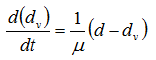The value of the viscous damage (dv) can be calculated from the current damage (d), the viscosity parameter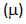, and the time step used in the analysis (dt).  The viscous damage value is then substituted for the regular damage value to calculate updated tractions, and is also used to update the tangent stiffness matrix.  With a small value of the viscosity parameter, the rate of convergence is improved without compromising the results.  In addition, the viscous energy dissipation is usually available as a result quantity so that the analyst can compare to the strain energy to verify that this damping procedure is not adding artificial stiffness to the system.

To illustrate, consider the double cantilever beam specimen in Figure 2, modeled using 2D plane strain elements and cohesive zone interface elements, where the interface is to be separated by displacing the ends by 4mm in both the up and down directions.  Even for this simple problem with 200 elements along the length and 4 through the thickness, a converged solution was not obtained even when breaking up the loading into 10,000 substeps.

Figure 2: Geometry of Double Cantilever Beam Specimen

## czm2.pngThree additional analyses were run, using viscosity regularization with viscosity values of 1.0, 0.1 and 0.01. Figure 3 contains the force-displacement results for the loaded end of the specimen.  The larger values of viscosity over-estimate the positive tangent stiffness effect, since it is expected that the bilinear interface law behavior will show a sharp peak at damage initiation.  Guidelines for verifying a valid solution would include further reduction of the viscosity value until the change in response is insignificant, and reviewing the viscous energy dissipation to determine if it is a negligible percentage of the strain energy in the system. As with any numerical damping scheme, the lower the viscosity value, the more accurate the results, the lower the viscous energy, but the longer the analysis run time.

Figure 3: Force-Displacement Plots Comparing Different Viscosity Parameter Values

## czm3.pngThe delamination of the specimen at the end of the loading is shown in Figure 4.

Figure 4: Displacement Contour Plot of Delamination Specimen

## czm4.pngThe use of viscous regularization is a powerful and often necessary tool that enables accurate prediction of delamination when used with the bilinear cohesive zone model but needs to be carefully verified as illustrated above.  Let me know if you have used viscous regularization and have had success with it.